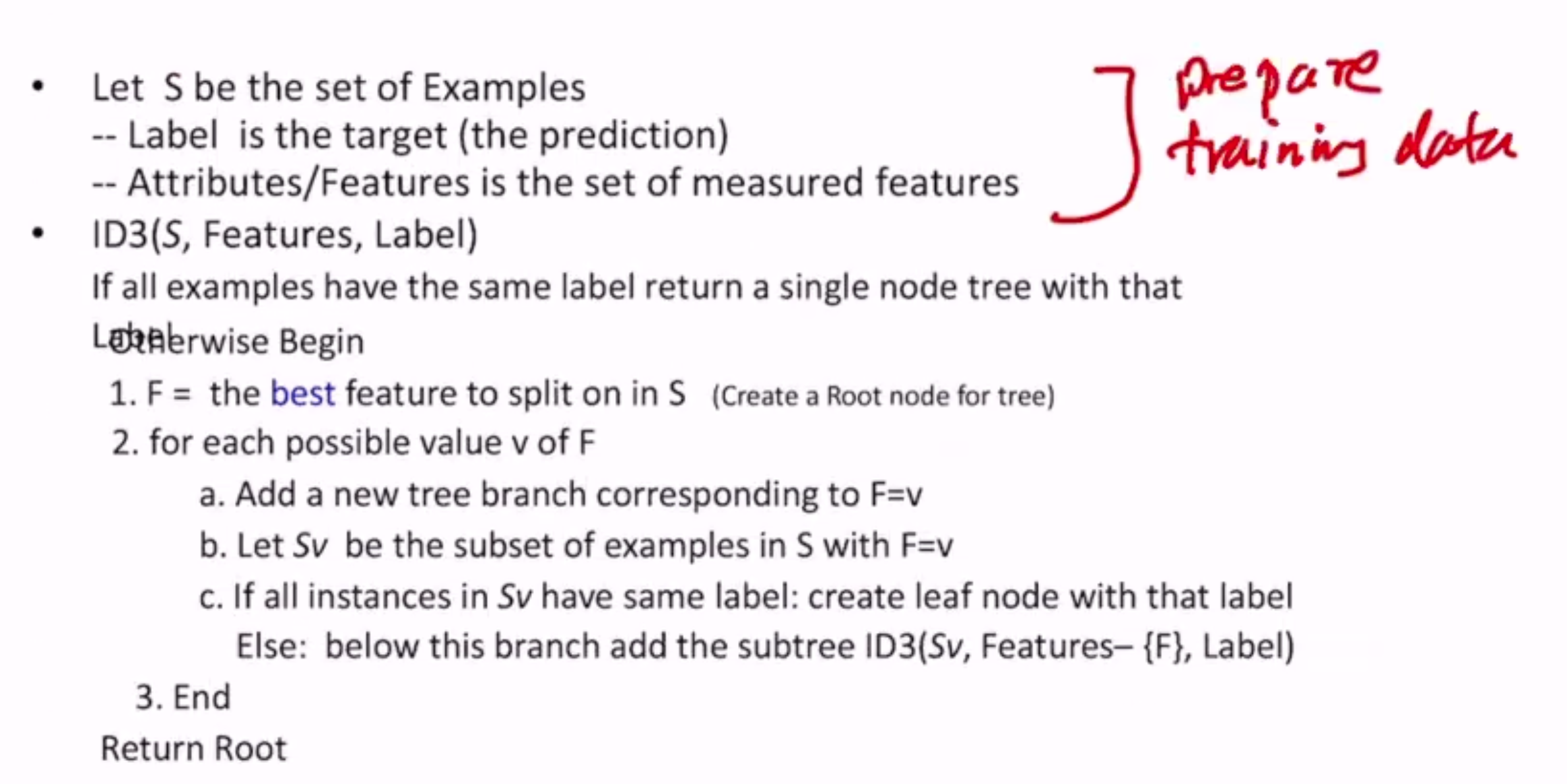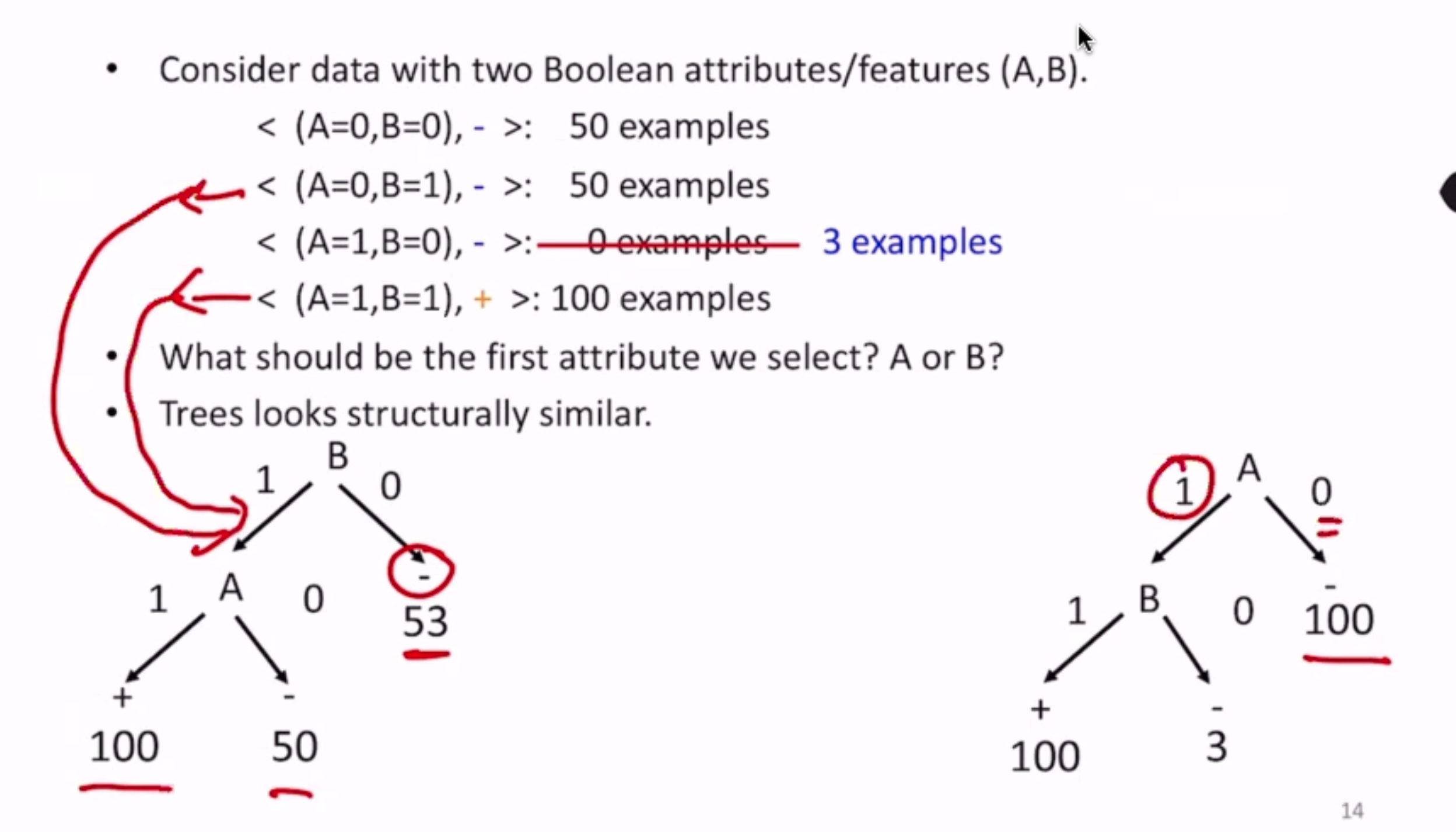# Decision Trees¶

Let’s take the example of whether or not to play tennis given 4 features - a binary classification question based on discrete features.

To construct, pick a feature and split on it - then recursively build the tree top down

## Algorithm¶

ID3 = Iterative DichotomizerHow do we choose the “best” feature?

• Our goal is to build a tree that is as small as possible - Occam’s Razor
• select a hypothesis that makes minimal assumptions
• finding the minimal tree is NP-hard
• this algorithm is greedy-heuristic, but cannot guarantee optimality
• the main heuristic is the definition of “best”

## “Best”¶

### Example¶

Take the dataset:

(A=0,B=0), -: 50 examples
(A=0,B=1), -: 50 examples
(A=1,B=0), -: 0 examples
(A=1,B=1), +: 100 examples


In this case, it’s easy to tell we should split on A.We prefer the right tree (split on A) because statistically it requires fewer decisions to get to a prediction. But how do we quantify this?

We should choose features that split examples into “relatively pure” examples. This is based on information gain.

### Entropy¶

Entropy of a set of examples S relative to a binary classification task is: (also denoted $$H(S)$$)

$Entropy(S) = -p_+ \log_2(p_+) - p_- \log_2(p_-)$

Where $$p_+$$ is the proportion of examples in S that belong to positive class, and vice versa

Generalized to K classes:

$Entropy(S) = \sum_{i=1}^K -p_i \log_2(p_i)$

The lower the entropy, the less uncertainty there is in a dataset.

Ex. When all examples belong to one class, entropy is 0. When all examples are exactly split across all classes (in binary case), entropy is 1.

### Information Gain¶

The information gain of an attribute $$a$$ is the expected reduction in entropy caused by partitioning on this attribute:

$Gain(S, a) = Entropy(S) - \sum_{v \in values(a)} \frac{|S_v|}{|S|}Entropy(S_v)$

where $$S_v$$ is the subset of a for which the value of attribute $$a$$ is $$v$$.

This takes the averaged entropy of all partitions, and the entropy of each partition is weighted by its “contribution” to the size of S.

Partitions of low entropy (low uncertainty/imbalanced splits) result in high gain

So this algorithm (ID3) makes statistics-based decisions that uses all data

## Overfitting¶

• Learning a tree that perfectly classifies the data may not create the tree that best generalizes the training data
• may be noise
• might be making decisions based on very little data
• causes:
• too much variance in training data
• we split on features that are irrelevant

### Pruning¶

Pruning is a way to remove overfitting

• remove leaves and assign majority label of parent to all items
• prune the children of S if:
• all children are leaves
• how much to prune?
• prune if the accuracy on the validation set does not decrease upon assigning the most frequent class label to all items at S

2 basic approaches:

• post-pruning: grow the full tree then remove nodes that don’t have sufficient evidence
• pre-pruning: stop growing the tree at some point during construction when it is determined that there is not enough data to make reliable choices

Note that not all leaves in a pruned tree have to be pure. How do we decide the label at a non-pure leaf?

### Random Forests¶

Another way to avoid overfitting

• learn a collection of decision trees instead of a single one
• predict the label by aggregating all the trees in the forest
• voting for classification, averaging for regression

## Continuous Attributes¶

TLDR:

• split on feature that gives largest drop in MSE
• split continuous variables into discrete variables (small, medium, large, etc)
• what thresholds to use for these splits?
• e.g. sort examples on feature, for each ordered pair with different labels consider if there is a threshold
• midpoint or on the endpoint?

## Missing Values¶

Options:

• throw away the instance
• fill in the most common value of the feature
• fill in with all possible values of the feature
• guess based on correlations with other features/labels

## Other Issues¶

• noisy labels
• two identical instances with conflicting labels
• attributes with different costs
• change information so that low cost attributes are preferred